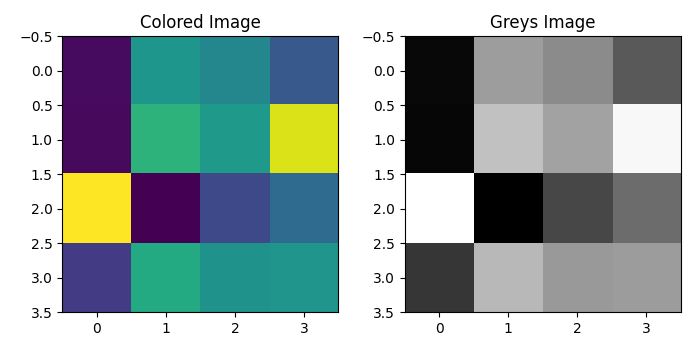# Plot a black-and-white binary map in Matplotlib

To plot black-and-white binary map in matplotlib, we can create and add two subplots to the current figure using subplot() method, where nrows=1 and ncols=2. To display the data as a binary map, we can use greys colormap in imshow() method.

## Steps

• Create data using numpy
• Add two sublots, nrows=1 and ncols=2. Consider index 1.
• To show colored image, use imshow() method.
• Add title to the colored map.
• Add a second subplot at index 2.
• To show the binary map, use show() method with Greys colormap.
• To adjust the padding between and around the subplots, use tight_layout() method.
• To display the figure, use show() method.

## Example

import numpy as np
from matplotlib import pyplot as plt
plt.rcParams["figure.figsize"] = [7.00, 3.50]
plt.rcParams["figure.autolayout"] = True
data = np.random.rand(4, 4)
plt.subplot(121)
plt.imshow(data)
plt.title("Colored Image")
plt.subplot(122)
plt.imshow(data, cmap='Greys_r', interpolation='nearest')
plt.title("Greys Image")
plt.tight_layout()
plt.show()

## Output